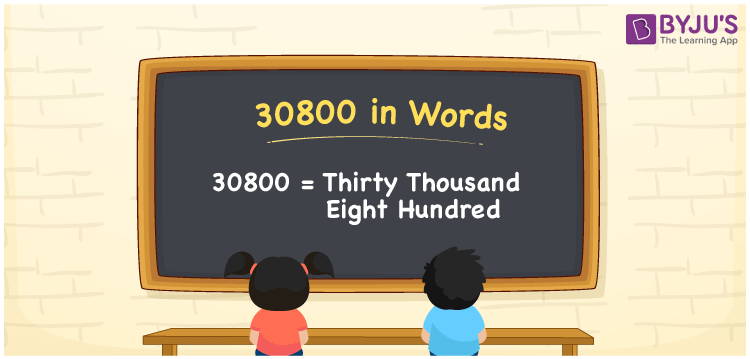# 30800 in Words

30800 in words is written as “Thirty Thousand Eight Hundred”. 30800 is equal to 30000 + 800. It can be represented in various other forms. For example, Rs. 30800 is given by Rupees “Thirty Thousand Eight Hundred only” in a bank cheque. In Mathematics, 30800 is also a cardinal number, that is to represent the quantity or value of something. Let us see in this article how to write 30800 in words using place value method.

 30800 in Words Thirty Thousand Eight Hundred Thirty Thousand Eight Hundred in Numbers 30800

## 30800 in English Words## How to Write 30800 in Words?

30800 is a five-digit number that can be written in words, by knowing the position of each digit in the number. This can be done using a place value chart.

 Ten thousands Thousands Hundreds Tens Ones 3 0 8 0 0

We can see, from the above table, the positions of digits are:

• 3 → Ten thousands
• 0 → Thousands
• 8 → Hundreds
• 0 → Tens
• 0 → Ones

Hence, when we read the number from right to left, it is given by Thirty Thousand Eight Hundred. Learn more about numbers in words at BYJU’S.

### Expanded Form of 30800

We can write the expanded form as:

3 × Ten thousand + 0 × Thousand + 3 × Hundred + 0 × Ten + 0 × One

= 3 × 10000 + 0 × 1000 + 8 × 100 + 0 × 10 + 0 × 1

= 30000 + 0 + 800 + 0 + 0

= 30800

= Thirty Thousand Eight Hundred

30800 is the natural number that is succeeded by 30799 and preceded by 30801. Learn more about the number 30800 below:

• 30800 in Words – Thirty Thousand Eight Hundred
• Is 30800 an odd number? – No
• Is 30800 an even number? – Yes
• Is 30800 a perfect square number? – No
• Is 30800 a perfect cube number? – No
• Is 30800 a prime number? – No
• Is 30800 a composite number? – Yes

## Frequently Asked Questions on 30800 in words

Q1

### What is 30800 in words?

30800 in words is given by Thirty Thousand Eight Hundred.
Q2

### What is the place value of 8 in 30800?

The place value of 8 in 30800 is Hundreds.
Q3

### How to spell 30800 in English words?

30800 in English is spelt as Thirty Thousand Eight Hundred